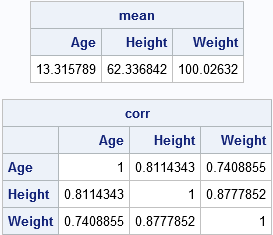In the SAS/IML language, you can read data from a SAS data set into a set of vectors (each with their own name) or into a single matrix. Beginning programmers might wonder about the advantages of each approach. When should you read data into vectors? When should you read data into a matrix?

### Read data into SAS/IML vectors

You can specify the names of data set variables in the SAS/IML READ statement, as follows:

```proc iml; use Sashelp.Class; /* open the data set */ read all var {"Name" "Height" "Weight"}; /* read 3 vars into vectors */ close Sashelp.Class; /* close the data set */```

The previous statements create three vectors, whose names are the same as the variable names. You can perform univariate analyses on the vectors, such as descriptive statistics. You can also create new variables from arbitrary transformations of the vectors, such as the following computation of the body mass index:

```BMI = weight / height##2 * 703; print BMI[rowname=Name];```• Variables are given informative names.
• You can use a single READ statement to read character variables and numerical variables.

When you load summarized data, you might want to read the variables into vectors. For example, to read the ParameterEstimates table from a regression analysis, you probably want to read the variable names, parameter estimates, standard errors, and p-values into separate vectors.

### Read data into a SAS/IML matrix

You can use the INTO clause in the READ statement to read data set variables into a SAS/IML matrix. All the variables have to be the same type, such as numeric. For example, the following statements read three numeric variables into a matrix:

```proc iml; varNames = {"Age" "Height" "Weight"}; /* these vars have the same type */ use Sashelp.Class; /* open the data set */ read all var varNames into X; /* read 3 vars into matrix */ close Sashelp.Class; /* close the data set */```

The matrix X contains the raw data. Each column is a variable; each row is an observation. For many descriptive statistics, you can use a single function call to compute statistics across all columns. You can also compute multivariate statistics such as a correlation matrix:

```mean = mean(X); /* mean of each column */ corr = corr(X); /* correlation matrix */ print mean[colname=varNames], corr[colname=varNames rowname=varNames];```You can use this technique to create vectors whose names are different from the names of data set variables. For example, in my blog posts I often load data into vectors named x and y to emphasize that the subsequent analysis will work for any data, not just for the example data.

• You can compute descriptive statistics for all columns by using a single function call.
• You can sort, transpose, or reorder columns of the data.
• You can compute row operations, such as the sum across rows.
• You can compute multivariate statistics such as finding complete cases or computing a correlation matrix.
• Many statistical analyses, such as least squares regression, have a natural formulation in terms of matrix operations.

I usually read raw data into a matrix and summarized data into vectors, but as you can see, there are advantages to both approaches.

What technique do you use to read data into SAS/IML?

Share